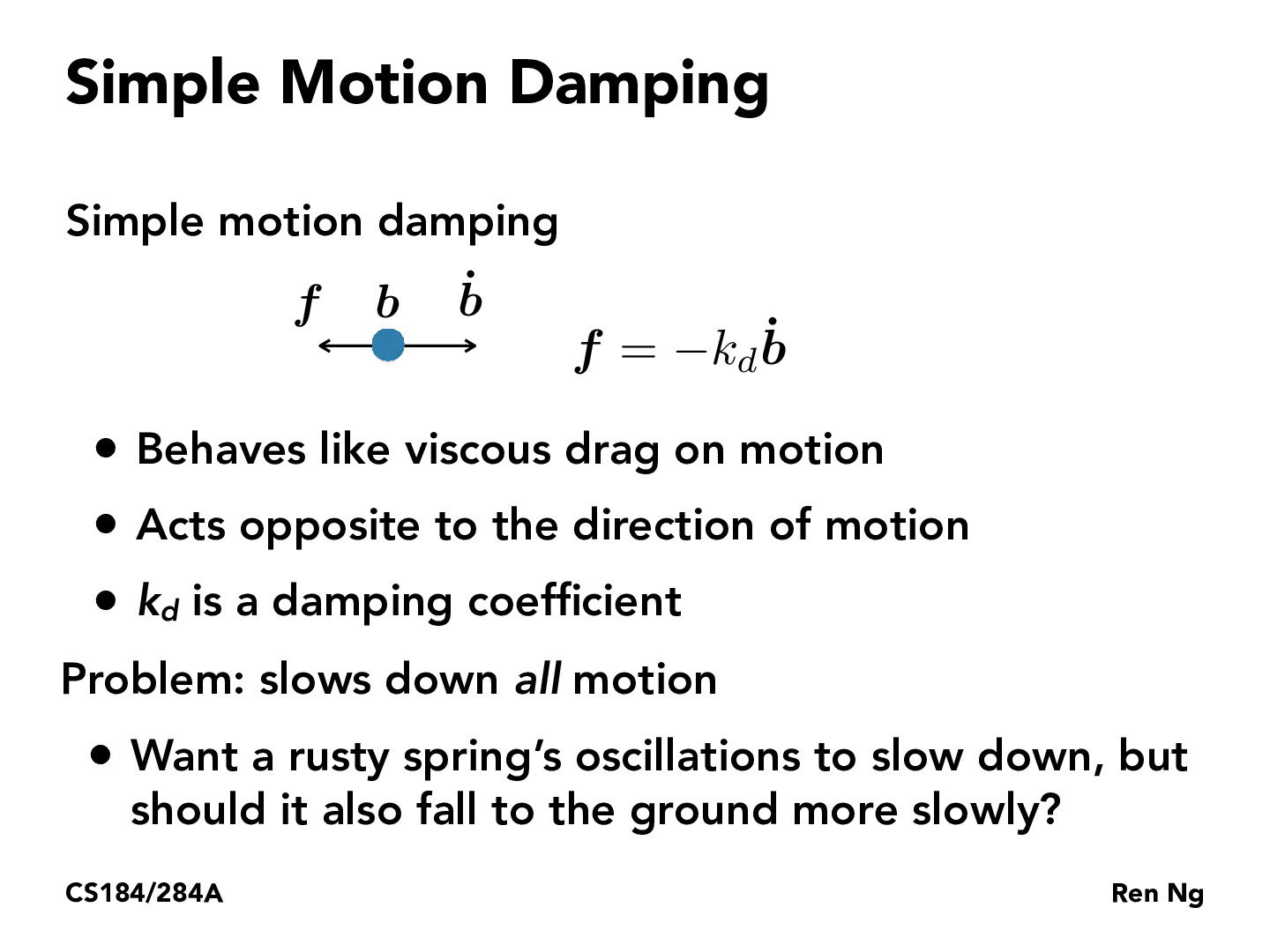You are viewing the course site for a past offering of this course. The current offering may be found here.Lecture 18: Physical Simulation (16)sunsarah

How is the damping coefficient calculated? Do you just try a bunch of coefficients and then see which one models whatever is trying to be modeled the most accurately? Is there a more algorithmic way to find it?jenzou

@sunsarah I found these websites:

https://en.wikipedia.org/wiki/Damping_ratio#Derivation

http://hyperphysics.phy-astr.gsu.edu/hbase/oscda.html#c2

Undamped ($\zeta$ = 0), underdamped ($\zeta$ < 1), critically damped ($\zeta$ = 1), overdamped ($\zeta$ > 1)zsano1

Why we have velocity here? According to Hooke's law, isn't it the distance to the balance position?armantark

Didn't we assign the single dot to mean derivative? What does it mean to derive a vector? Wouldn't it just compute to the zero vector due to all the values being (presumably) constants or am I missing something?

You must be enrolled in the course to comment